# Cluster Data

Cluster data using k-means algorithm in the Live Editor

Since R2021b

## Description

The Cluster Data Live Editor Task enables you to interactively perform k-means clustering. The task generates MATLAB® code for your live script and returns the resulting cluster indices and the cluster centroid locations to the MATLAB workspace.

You can:

• Determine the optimal number of clusters for your data manually by selecting the number of clusters or automatically by specifying criteria such as gap values, silhouette values, Davies-Bouldin index values, and Calinski-Harabasz index values.

• Customize the parameters for clustering your data, including the distance metric and the number of replicates.

• Automatically visualize the clustered data.

• On the Live Editor tab, select Task > Cluster Data.

• In a code block in the live script, type a relevant keyword, such as `clustering` or `kmeans`. Select Cluster Data from the suggested command completions.

## Examples

expand all

This example shows how to use the Cluster Data task to interactively perform k-means clustering for a specified number of clusters.

Load the sample data. The data contains length and width measurements from the sepals and petals of three species of iris flowers.

`load fisheriris`

Open the Cluster Data task. To open the task, begin typing the keyword `clustering` in a code block and select Cluster Data from the suggested command completions.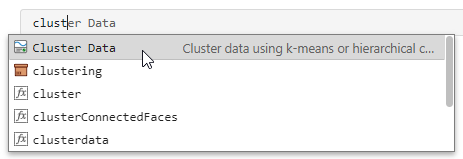Cluster the data into two clusters.

• Select the meas variable as the input data.

• Set the number of clusters to `2`.

• In the Live Editor tab, press the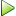button to run the task.

MATLAB displays the clustered data and the cluster means in a scatter plot.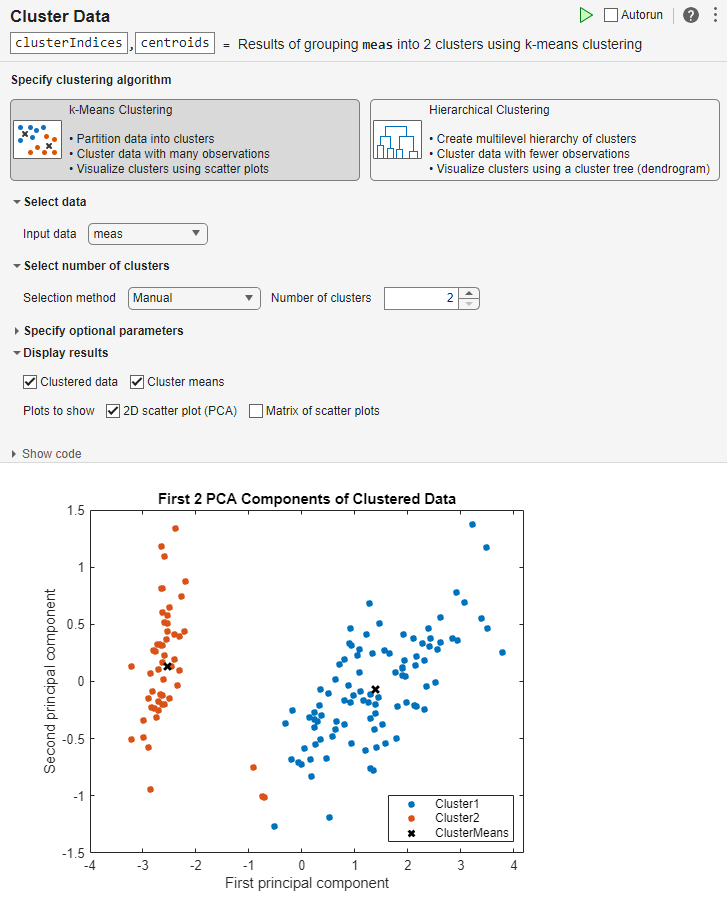Increase the number of clusters to `3` and rerun the task. MATLAB displays the updated clustered data and the cluster means in a scatter plot.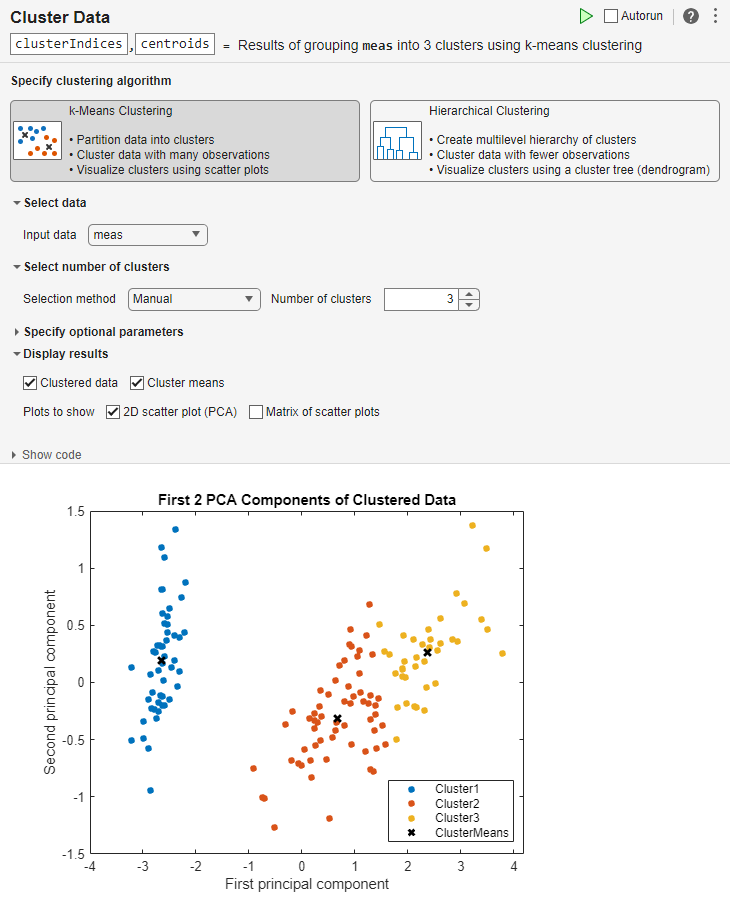The task generates code in your live script. The generated code reflects the parameters and options that you select, and includes code to generate the scatter plot. To see the generated code, click theat the bottom of the task parameter area. The task expands to display the generated code.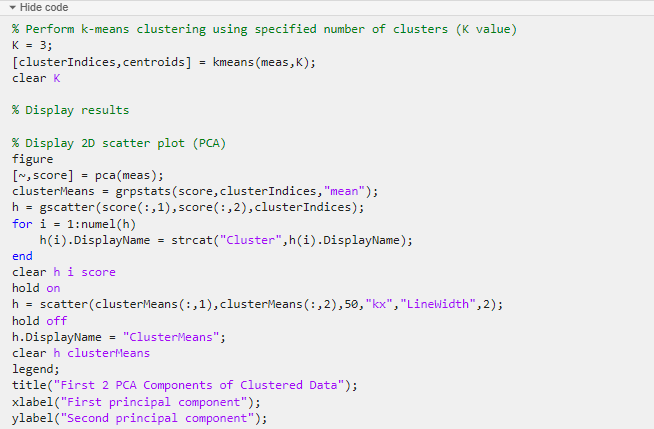By default, the generated code uses `clusterIndices` and `centroids` as the name of the output variables returned to the MATLAB workspace. The `clusterIndices` vector is a numeric column vector containing the cluster indices. Each row in `clusterIndices` indicates the cluster assignment of the corresponding observation. The `centroids` matrix is a numeric matrix containing the cluster centroid locations. To specify a different output variable name, enter a new name in the summary line at the top of the task. For instance, change the two variable names to `c_indices` and `c_locations`.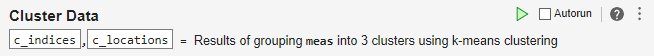When the task runs, the generated code is updated to reflect the new variable names. The new variables `c_indices` and `c_locations` appear in the MATLAB workspace.

This example shows how to use the Cluster Data task to interactively evaluate clustering solutions based on selected criteria.

Load the sample data. The data contains length and width measurements from the sepals and petals of three species of iris flowers.

`load fisheriris`

Open the Cluster Data task. To open the task, begin typing the keyword `clustering` in a code block and select Cluster Data from the suggested command completions.Evaluate the optimal number of clusters.

• Select the meas variable as the input data.

• Set the number of clusters selection method to `Optimal`.

• Set the range min and max to `2` and `6`.

• In the Live Editor tab, press thebutton to run the task.

MATLAB displays a bar chart with evaluation results, indicating that, based on the Calinski-Harabasz criterion, the optimal number of clusters is 3. A scatter plot shows the clustered data and the cluster means using the optimal number of clusters, 3. Your results may differ.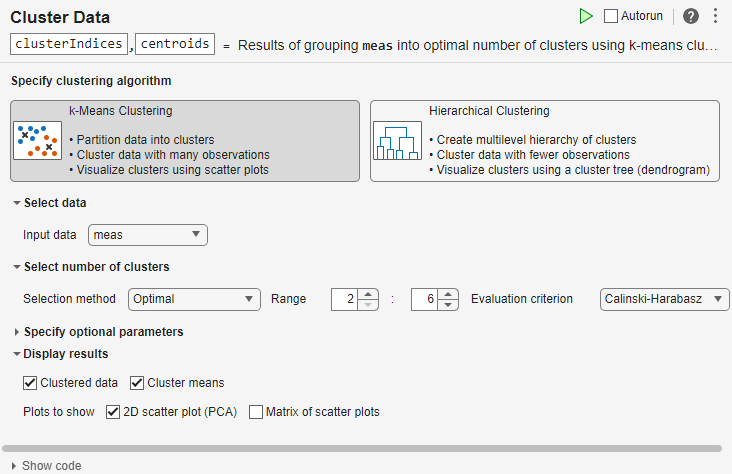## Parameters

expand all

Specify the data to cluster by selecting a variable from the available workspace variables. The variable must be a numeric matrix to appear in the list.

Specify the method for determining the optimal number of clusters for your data.

• `Manual` — Specify the number of clusters to group your data into manually.

• `Optimal`— Use the `evalclusters` function to find the optimal number of clusters based on criteria such as gap values, silhouette values, Davies-Bouldin index values, and Calinski-Harabasz index values.

Specify the list of number of clusters to evaluate as a range consisting of a min value and a max value. For example, if you specify a min value of `2` and a max value of `6`, the task evaluates the number of clusters 2, 3, 4, 5, and 6 to determine the optimal number.

To display the clustered data, select from the available options:

• Select 2D scatter plot (PCA) to display the principle components of the clustered data in a 2D scatter plot. The Cluster Data task uses the `gscatter` function to create the scatter plot.

• Select Matrix of scatter plots to display the clustered data in a matrix of scatter plots. When you select Matrix of scatter plots, a list appears to the right of the check box. Each item in the list represents a column in the specified input data. Press the Ctrl key and select a maximum of four input data columns from the list. The Cluster Data task uses the `pca` and `gplotmatrix` functions to create the matrix of scatter plots from the selected columns.

The scatter plots in the matrix compare the selected input data columns across cluster indices. The diagonal plots in the matrix are histograms showing the distribution of the selected columns for each cluster indices.

## Tips

• By default, the Cluster Data task does not automatically run when you modify the task parameters. To have the task run automatically after any change, select the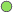button at the top-right of the task. If your dataset is large, do not enable this option.

## Version History

Introduced in R2021b